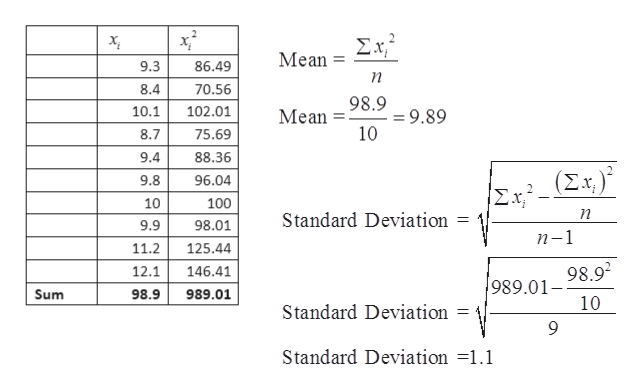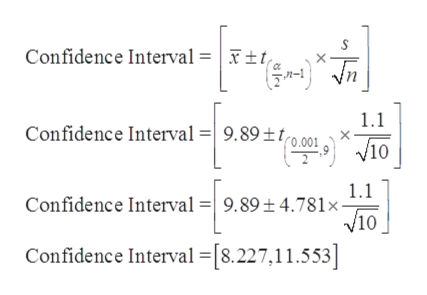# Over the past several months, an adult patient has been treated for tetany (severe muscle spasms). This condition is associated with an average total calcium level below 6 mg/dl. Recently, the patient's total calcium tests gave the following readings (in mg/dl). Assume that the population of x values has an approximately normal distribution.9.38.410.18.79.49.810.09.911.212.1(a) Use a calculator with mean and sample standard deviation keys to find the sample mean reading x and the sample standard deviation s. (Round your answers to two decimal places.)x = mg/dls = mg/dl(b) Find a 99.9% confidence interval for the population mean of total calcium in this patient's blood. (Round your answer to two decimal places.)lower limit     mg/dlupper limit     mg/dl(c) Based on your results in part (b), do you think this patient still has a calcium deficiency? Explain.Yes. This confidence interval suggests that the patient may still have a calcium deficiency.Yes. This confidence interval suggests that the patient no longer has a calcium deficiency.No. This confidence interval suggests that the patient may still have a calcium deficiency.No. This confidence interval suggests that the patient no longer has a calcium deficiency.

Question
1764 views

Over the past several months, an adult patient has been treated for tetany (severe muscle spasms). This condition is associated with an average total calcium level below 6 mg/dl. Recently, the patient's total calcium tests gave the following readings (in mg/dl). Assume that the population of x values has an approximately normal distribution.

 9.3 8.4 10.1 8.7 9.4 9.8 10 9.9 11.2 12.1
(a) Use a calculator with mean and sample standard deviation keys to find the sample mean reading x and the sample standard deviation s. (Round your answers to two decimal places.)
 x = mg/dl s = mg/dl

(b) Find a 99.9% confidence interval for the population mean of total calcium in this patient's blood. (Round your answer to two decimal places.)
 lower limit mg/dl upper limit mg/dl

(c) Based on your results in part (b), do you think this patient still has a calcium deficiency? Explain.
Yes. This confidence interval suggests that the patient may still have a calcium deficiency.
Yes. This confidence interval suggests that the patient no longer has a calcium deficiency.
No. This confidence interval suggests that the patient may still have a calcium deficiency.
No. This confidence interval suggests that the patient no longer has a calcium deficiency.
check_circle

Step 1

In this question, we have the patients calcium reading, using that we have to find the Mean, Standard deviation, then we have to find the 99.9% confidence interval and then we have to draw the conclusion.

Step 2

The calculations for mean and standard deviation have shown below.help_outlineImage TranscriptioncloseΣΧ Mean 9.3 86.49 n 8.4 70.56 98.9 =9.89 10 10.1 102.01 Mean 8.7 75.69 9.4 88.36 (Σ) 9.8 96.04 Σx. 10 100 Standard Deviation 98.01 9.9 n-1 11.2 125.44 98.92 989.01- 10 12.1 146.41 Sum 98.9 989.01 Standard Deviation 9 Standard Deviation =1.1 fullscreen
Step 3

The calculations for the confidence inter...help_outlineImage TranscriptioncloseConfidence Interval = | x ±t} * {n | Confidence Interval =| 9.89 ±tro.001*10 1.1 1.1 Confidence Interval =| 9.89± 4.781x- V10 Confidence Interval =[8.227,11.553] fullscreen

### Want to see the full answer?

See Solution

#### Want to see this answer and more?

Solutions are written by subject experts who are available 24/7. Questions are typically answered within 1 hour.*

See Solution
*Response times may vary by subject and question.
Tagged in

### Hypothesis Testing